# Distance of Earth-Sun

Hello all!
Who can explain under what formula the calculated value of the distance Earth-Sun
in the metadata file S2A MSI L1C product?
Why ask this question? For example, for the S2A data from 3.01.2016 this value
is: 1.03423414807786
However, in such sources as:

1. Mather, Paul M. Computer processing of remotely-sensed images: an introduction / Paul M. Mather and Magaly Koch. – 4th ed.
Page 120;
2. Applications of satellite and airborne image data to coastal management. Seventh computer-based learning module (third edition) (revised and expanded for BILKO 3) Edwards, A.J. (Ed.) (2005). Applications of satellite and airborne image data to coastal management. Seventh computer-based learning module (third edition) (revised and expanded for BILKO 3). Coastal Region and Small Island Papers, 18. UNESCO: Paris. vi, 242 + appendices pp.
http://www.vliz.be/imisdocs/publications/270191.pdf
Page 136
Given the formula:

d = 1-0.01674COS(PI(360/365.256363)*(JDAY-4)/180);

The values presented in table 11.4 on page 119 in
Landsat Science Data Users Handbook
http://landsathandbook.gsfc.nasa.gov/pdfs/Landsat7_Handbook.pdf
or in the file:
http://landsathandbook.gsfc.nasa.gov/excel_docs/d.xls
When computing both the formula and the table comes out a different value.
Using the source program GRASS GIS with a slightly different approach, we obtain approximately the same value.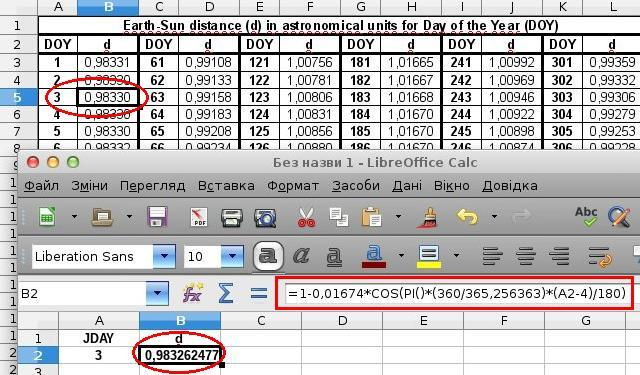Why in the metadata file specified 1.03423414807786?

Best Regards,
Igor.

Hi Igor,

The TOA Reflectance Computation is calculated using Equation 1 here:

https://sentinel.esa.int/web/sentinel/technical-guides/sentinel-2-msi/level-1c/algorithm

It uses Equation 2 as the calculation for d(t) - the correction factor accounting for the Earth-Sun distance

Cheers

Jan

1 Like

Somehow I missed this formula. But the issue remained. Why S2A different method of calculation? Why in the formula has the value of 0.0172 (the Earth angular velocity (radians/day))? All other methods were wrong? They give a completely different result.

Sorry! Already understand: 0.0172*180/PI ~ 0.9855 <=> 360/365.256363
Ok.
The issue has been resolved. But then the question: why “(t-2)”?

Hi Igor,

It’s a good question, and one that I cannot answer, currently. I could ask:

Why (JDAY-4) in BILKO?I’ll keep digging through the documentation.

Cheers

Jan

S2 MPC Operations Manager

1 Like

Ok. The issue is resolved:
d(t) = 1/ ((1-0.01673cos(0.0172(t-2)))^2) = 1/d^2
d(t) – this is value from metadata!
Distance of Earth-Sun: d = SQRT(1/d(t)).

Thank you.

2 Likes

Can you please explain how to get the values to calculate the TOA or BOA reflectance ?
L = (rToa * e0__SOLAR_IRRADIANCE_For_band * cos(Z__Sun_Angles_Grid_Zenith_Values)) / (PI * U__earth_sun_distance_correction_factor) (Equation 1)
I know that :
rToa is the band we need to calculate its reflectance
e0__SOLAR_IRRADIANCE_For_band can be obtained from the metadata as below for Band 4 for example :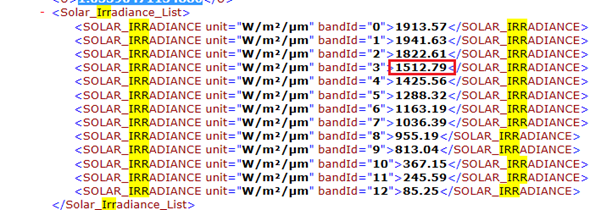U__earth_sun_distance_correction_factor from the metadata as below :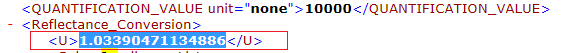But how to obtain:
1- cos(Z__Sun_Angles_Grid_Zenith_Values))
2- PI ( what is it ?)

I have calculated the d(t) according to the equation :As t= 0.98358 (for the day for day 358, the earth-sun distance is 1.00992).
d(t) = 1.03431890515045328251315
But do not know where to insert d(t) in the (Equation 1)

Another question regarding :
(Equation 2)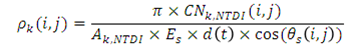Another question, why using the Equation 1 and not Equation 2 for calculating the reflectance? Also would be more appreciate if you could give an example of how to fine the parameters in Equation 2 from Sentinel 2 metadata.

Thanks a lot for your help and time,
D

Hi, Daniel

Data Sentinel-2A L1C MSI already have TOA Reflectance. According to the documentation, You must divide the pixel value by the value of QUANTIFICATION_VALUE:

rTOA = DN / QUANTIFICATION_VALUE

Possible also some deviation in DN from the desired range. For example:

For BOA Reflectance – use, for example, SEN2COR package:

L – this is the TOA spectral radiance – the formula is the reverse formula for finding TOA spectral reflectance. For example: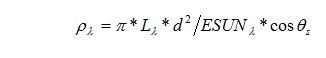http://yceo.yale.edu/how-convert-landsat-dns-top-atmosphere-toa-reflectance

d = SQRT(1/d(t)) or d =SQRT(1/U)
Theta = Z__Sun_Angles_Grid_Zenith_Values – the interpolated value from the matrix from the metadata:
GRANULE \ … granule.xml, tags: “Tile_Angles Sun_Angles_Grid Zenith… Values_List…”

1 Like

L = (rToa * e0__SOLAR_IRRADIANCE_For_band * cos(Z__Sun_Angles_Grid_Zenith_Values)) / (PI * U__earth_sun_distance_correction_factor)

Please note that earth_sun_distance_correction_factor – this value U.

1 Like

@Igor thanks a lot for the reply and for the explanation.
Just still on question: what is PI in L equation ?

L = (rToa * e0__SOLAR_IRRADIANCE_For_band * cos(Z__Sun_Angles_Grid_Zenith_Values)) /** (PI** * U__earth_sun_distance_correction_factor)

What is PI in L equation ?
PI ~3.141592 LikesThanks @Igor

The correct expression for the radiance should be the following:
L = (rToa * e0__SOLAR_IRRADIANCE_For_band * cos(Z__Sun_Angles_Grid_Zenith_Values) * U__earth_sun_distance_correction_factor) / PI
because U=1/d^2

1 Like

It’s the same formula!
Because:

rToa = (PILd^2)/(ESUNcos(theta));
L = (rToa
ESUNcos(theta))/(PId^2);
U=1/d^2;
d^2 = 1/U;
L = (rToaESUNcos(theta))/(PI/U);
L = (UrToaESUN*cos(theta))/PI;

1 Like

Now it is correct. In previous posts you’ve used the following expression:
L = (rToaESUNcos(theta))/(PI*U)I was misled by help to sen2three.
http://s2tbx.telespazio-vega.de/sen2three/html/r2rusage.html?highlight=quantification

But in the program sen2tree has the following lines:

===================

‘’’ Converts the reflectance to radiance.
:param indataArray: the digital numbers representing TOA reflectance.
:type indataArray: a 2 dimensional numpy array (row x column) of type unsigned int 16.
:return: the pixel data converted to radiance.
:rtype: a 2 dimensional numpy array (row x column) of type unsigned int 16, representing radiance.

``````        Additional inputs from L1 user Product_Image_Characteristics metadata:
* QUANTIFICATION_VALUE: the scaling factor for converting DN to reflectance.
* U: the earth sun distance correction factor.
* Sun_Angles_Grid.Zenith.Values: the interpolated zenith angles grid.
'''
# This converts TOA reflectance to radiance:
nrows = self.config.nrows
ncols = self.config.ncols
# The digital number (DN) as float:
DN = indataArr.astype(float32)
xp = L3_XmlParser(self.config, 'UP1C')
pic = xp.getTree('General_Info', 'Product_Image_Characteristics')
qv = pic.QUANTIFICATION_VALUE
c0 = 0

# The quantification value for the DN from metadata:
c1 =  float32(qv.text)

# TOA reflectance:
rtoa = float32(c0 + DN / c1)

rc = pic.Reflectance_Conversion

# The earth sun distance correction factor,
u2 =  float32(rc.U.text)

e0 = float32(si[self._bandIndex].text)

# The solar zenith array:
x = arange(nrows, dtype=float32) / (nrows-1) * self.config.solze_arr.shape
y = arange(ncols, dtype=float32) / (ncols-1) * self.config.solze_arr.shape
szi = rectBivariateSpline(x,y,self.config.solze_arr)
rtoa_e0_sza = float32(rtoa * sza * e0)
``````

???–>> pi_u2 = float32(pi * u2 )

``````    # Finally, calculate the radiance and return array as unsigned int, this is multiplied by 100,
# to keep resolution - glymur only allows integer integer values for storage.

L = (rtoa_e0_sza / pi_u2 ) * 100.0
return (L + 0.5).astype(uint16)
``````

===================

It seems to me that this formula is correct:
L = (UrToaESUN*cos(theta))/PI;
And in their program - error.

L = (UrToaESUN*cos(theta))/PI is correct expression, but you’ve written in other posts L = (rToa * e0__SOLAR_IRRADIANCE_For_band * cos(Z__Sun_Angles_Grid_Zenith_Values)) / (PI * U__earth_sun_distance_correction_factor)

perhaps it would be appropriate to correct1 Like

In sen2cor formula is correct!
Their review:
. . .

. . .

# scale: 1 / (0.001 * 1000) = 1 (default)

. . .
They use d^2:
L = (rToaESUNcos(theta))/(PI*d^2);